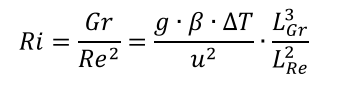# How EnergyPlus calculates Richardson number for zone flow regimeAccording to the Engineering reference of EnergyPlus, it calculates the Richardson number to determine the zone flow regime, thus determining which internal CHTC algorithm should be used during the simulation.

The Richardson number can be formulated by:Where Gr and Re mean the Grashof number and Reynolds number, respectively. g means the gravitational acceleration, β is the thermal expansion coefficient, ΔT is the temperature difference between surface and indoor air, u is the air velocity. LGr and LRe are the characteristic length for Gr and Re calculation.

The key point is which 'u' is used for Ri calculation? As the room air is usually assumed to be perfectly-mixed in EnergyPlus. Imagine that I have a mechanical ventilation system that supplies fresh air with 0.1 m3/s to the zone and it corresponds to about 10 air change rates per hour for the zone.

edit retag close merge delete